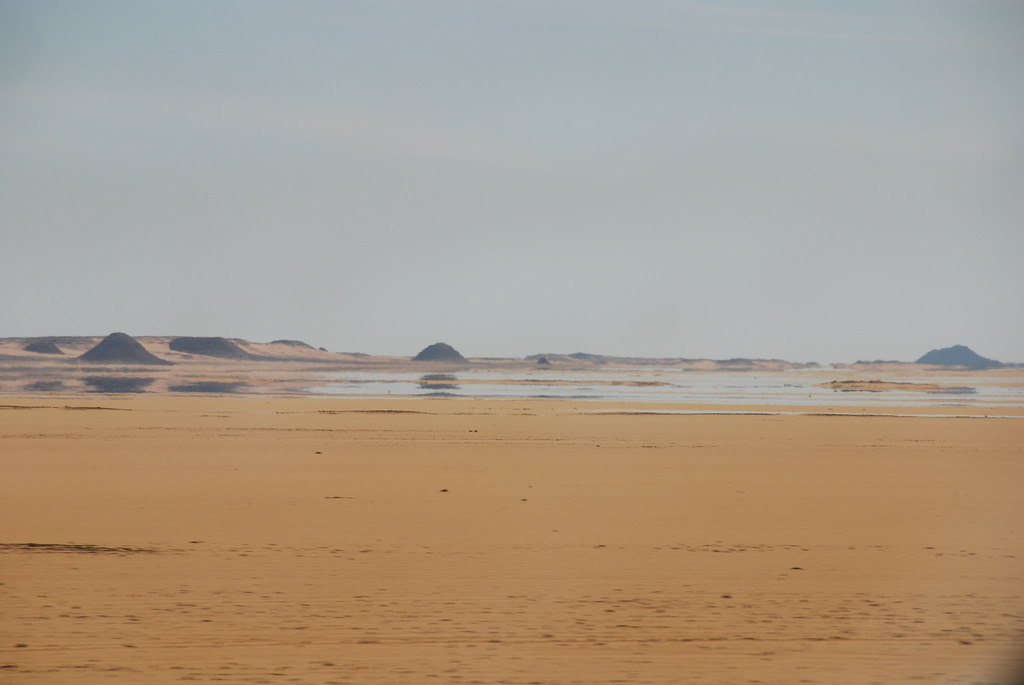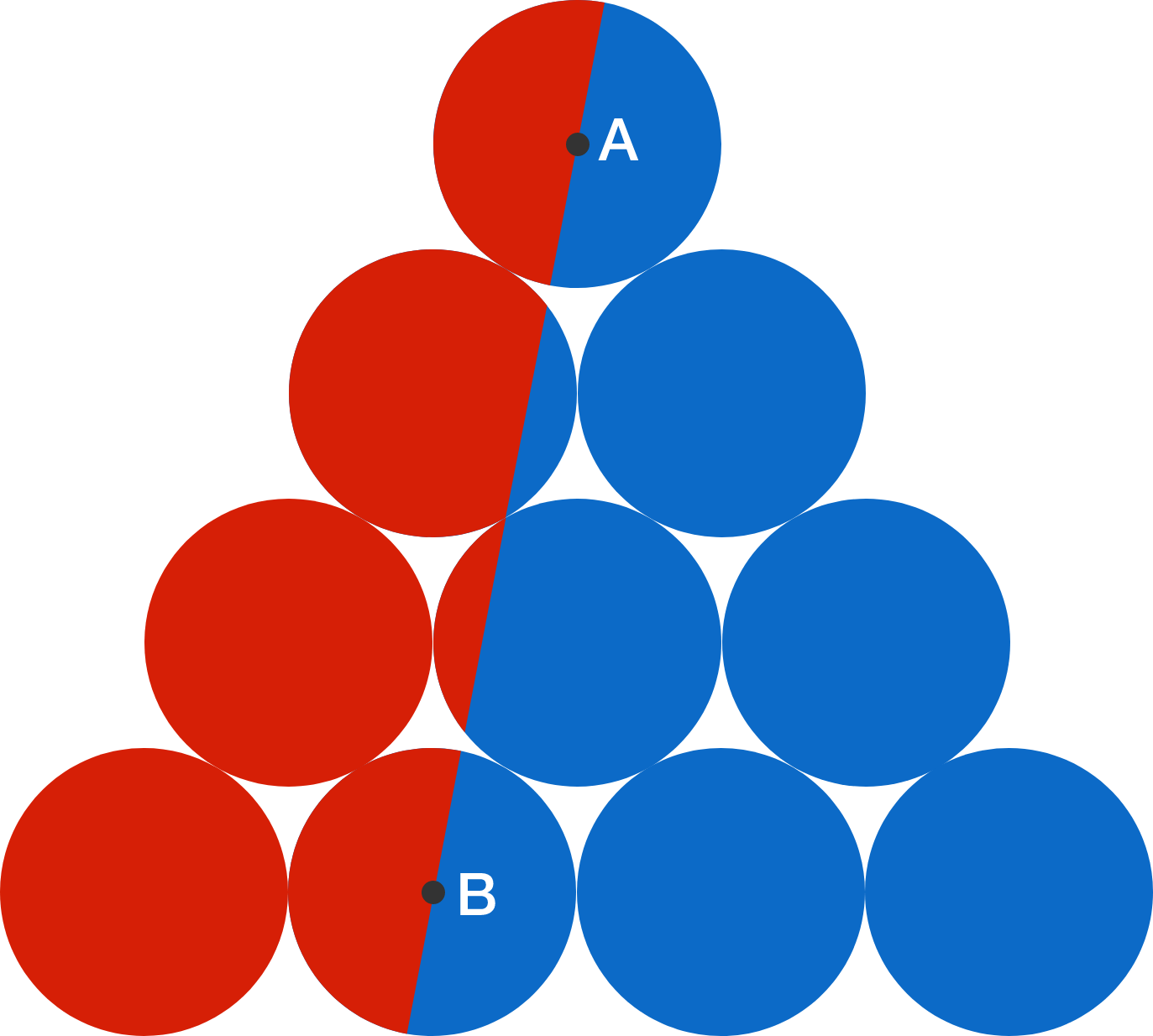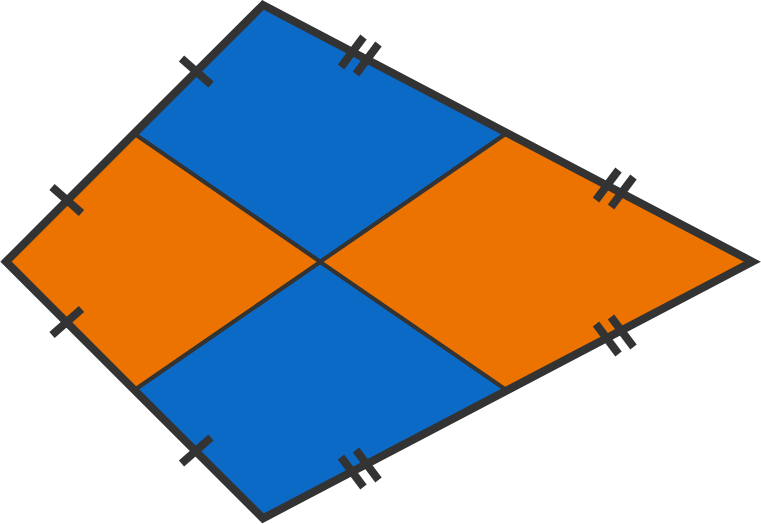# Problems of the Week

Contribute a problem

# 2017-11-20 Basic

A mirage is a frequent optical occurrence for desert travelers, which appears as a distant body of water. Which of the following best explains this phenomenon?Note: The mirage featured in this picture is known as an inferior mirage.

Alice arranges 3 coins in a row. You cannot see them, and need to guess the sequence—heads or tails—of the 3 coins. You make three guesses, and Alice responds as follows:Guess $\hspace{1.4cm}$ Response H H H "You got only 1 coin correct." T T H "You got only 1 coin correct." H T T "You got only 1 coin correct."

What is the correct sequence?

I'm thinking of a number.

• It is a two-digit positive integer.
• The sum of its digits is 10.
• Subtracting 72 from the number swaps its two digits.

What is the number?

10 identical circles are stacked in a pyramid shape, where $A$ and $B$ are centers of two of the circles. A line is drawn through $A$ and $B,$ dividing the figure into 2 areas: red on the left and and blue on the right.

What is the ratio of the red area to the blue area?A kite is divided into four regions by two line segments that connect the midpoints of opposite sides. Is the total blue area equal to the total orange area?×